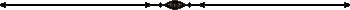Land  MeasurementsLink = 7.92 inches Rod = 16-1/2 feet = 1 Pole = 5-1/2 Yards = 25 Links Chain = 66 Feet = 4 Rods = 100 Links Furlong = 660 Feet = 40 Rods = 10 Chains Mile = 8 Furlongs = 320 Rods = 80 Chains = 5,280 Feet = 1,760 Yards Square Rod = 272-1/4 Square Feet = 30-1/4 Square Yards Acre = 10 Square Chains = 43,560 Square Feet = 160 Square Rods 1 Vara = 2.778 Feet = 33.33 Inches Foot = 12 Inches = .36 Vara Inch = .0833 Feet Arpent = 191.833 Feet = 30 Toise = 30 Compasses Square Arpent = 0.84628 Acres Acre = 5,645.376 Square Varas = 1.1834 Square Arpent Labor = 177.136 Acres = 1,000,000 Square Varas League = 4,428.4 Acres = 25,000,000 Square Varas. = 25 Labors